# Exercise E10.5 Circles NCERT Solutions Class 9

Go back to  'Circles'

## Chapter 10 Ex.10.5 Question 1

In the given figure, $$A,\, B$$ and $$C$$ are three points on a circle with center $$O$$ such that \begin{align}\angle {BOC}=30^{\circ} \text { and } \angle {AOB}=60^{\circ} \end {align}If  $$D$$ is a point on the circle other than the arc $$ABC,$$  find \begin{align} \angle {ADC} \end{align}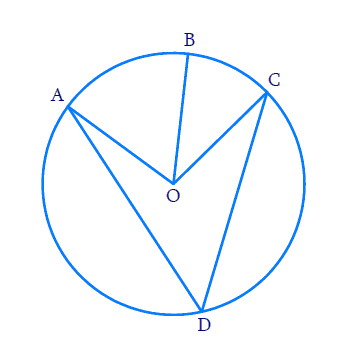### Solution

What is known?

Two angles subtended by arcs at the centre.

What is unknown?

Value of  $$\angle {ADC.}$$

Reasoning:

The angle subtended by an arc at the centre is double the angle subtended by it at any point on the remaining part of the circle.

Steps:

\begin{align} \angle {AOC} &=\angle {AOB}+\angle {BOC} \\ &=90^\circ \end{align}

By Theorem $$10.8$$ ,

\begin{align} \angle {AOC} &=2 \angle {ADC} \\ \angle ADC &= \frac{1}{2}\angle AOC\\\angle ADC &= \frac{1}{2} \times 90 = \,45^\circ \\\therefore \angle ADC &= \,45^\circ \end{align}

## Chapter 10 Ex.10.5 Question 2

A chord of a circle is equal to the radius of the circle. Find the angle subtended by the chord at a point on the minor arc and also at a point on the major arc.

### Solution

What is known?

Chord’s length is equal to the radius.

What is unknown?

Angle subtended by the chord at a point on the minor arc and also at a point on the major arc.

Reasoning:

• The angle subtended by an arc at the centre is double the angle subtended by it at any point on the remaining part of the circle.
• A quadrilateral $$ABCD$$ is called cyclic if all the four vertices of it lie on a circle.
• The sum of either pair of opposite angles of a cyclic quadrilateral is \begin{align}180^{\circ}.\end {align}

Steps: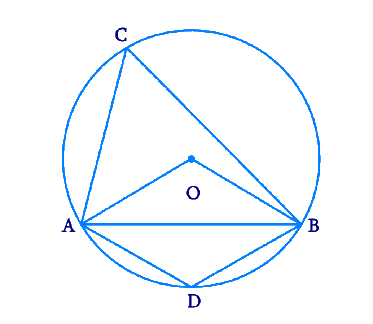Draw a circle with any radius and centre $$O$$. Let $$AO$$ and $$BO$$ be the $$2$$ radii of the circle and let $$AB$$ be the chord equal to the length of radius. Join them to form a triangle.

$$OA = OB = AB$$

Hence \begin{align} \Delta {ABO} \end {align} becomes an equilateral triangle.

Draw $$2$$ points $$C$$ and $$D$$ on the circle such that they lie on major arc and minor arc respectively.

Since \begin{align} \Delta {ABO} \end {align} is an equilateral triangle, we get \begin{align} \angle {ABO}=60^{\circ} \end {align}

For the arc $$AB,$$ \begin {align} \angle {AOB}=2 \angle {ACB} \end {align} as we know that the angle subtended by an arc at the centre is double the angle subtended by it at any point on the remaining part of the circle.

\begin{align}\angle ACB &= \frac{1}{2}\angle AOB\\&= \frac{1}{2} \times 60 \\&= 30^\circ \end{align}

As you can notice the points $$A, B, C$$ and $$D$$ lie on the circle. Hence $$A B C D$$ is a cyclic quadrilateral.

We know that, the sum of either pair of opposite angles of a cyclic quadrilateral is \begin{align}180^\circ.\end {align}

Therefore,

\begin{align}\angle ACB + \angle ADB &= 180^\circ \\30 + \angle ADB &= 180\\\angle ADB &= 150^\circ \end{align}

So when the chord of a circle is equal to the radius of the circle, the angle subtended by the chord at a point on the minor arc is \begin{align}150^\circ.\end {align} and also at a point on the major arc is \begin{align}30^\circ.\end {align}

## Chapter 10 Ex.10.5 Question 3

In the given figure, \begin {align} \angle {PQR}=100^{\circ} \end {align} where $$P, Q$$ and $$R$$  are points on a circle with center $$O$$ . Find \begin {align}\angle {OPR} \end {align}.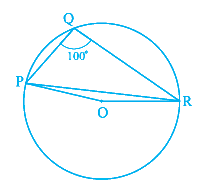### Solution

What is known?

$$3$$ points on the circle and

\begin {align}\angle {PQR}=100^{\circ} \end {align}

What is unknown?

Value of $$\angle {PQR}$$

Reasoning:

• The angle subtended by an arc at the centre is double the angle subtended by it at any point on the remaining part of the circle.
• A quadrilateral \begin {align} {ABCD} \end {align} is called cyclic if all the four vertices of it lie on a circle.
• The sum of either pair of opposite angles of a cyclic quadrilateral is \begin {align} 180^{\circ}. \end {align}

Steps:

Mark any point on the major arc side (opposite side to point \begin {align} {Q}\end {align}) as \begin {align} {S.}\end {align}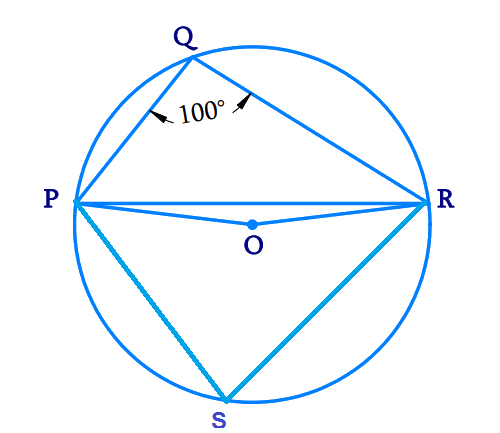Since all points \begin {align} {P, Q, R, S}\end {align} lie on the circle, \begin {align} {PQRS}\end {align} becomes a cyclic quadrilateral.

We know that, the sum of either pair of opposite angles of a cyclic quadrilateral is \begin {align} 180^\circ.\end {align}

Therefore,

\begin{align} \angle {PQR}+\angle {PSR} &=180^{\circ} \\ 100^{\circ}+\angle {PSR} &=180^{\circ} \\ \angle {PSR} &=180^{\circ}-100^{\circ} \\ &=80^{\circ} \end{align}

We know that, the angle subtended by an arc at the centre is double the angle subtended by it at any point on the remaining part of the circle.

Therefore,

\begin{aligned} \angle {POR} &=2 \angle {PSR} \\ &=2 \times 80^{\circ} \\ &=160^{\circ} \end{aligned}

Consider the \begin{aligned} \Delta {OPR.}\end{aligned} It is an isosceles triangle as

\begin{align} OP &= OR \\ &= \text{Radius of the circle.}\end{align}

\begin {align}∴ \angle {OPR}=\angle {ORP} \end {align}

Sum of all angles in a triangle is \begin {align}180^{\circ}. \end {align}

Therefore,

\begin{align}\angle {OPR}+ \!\angle {POR}+ \! \angle {ORP}&=180^{\circ} \\ \angle {OPR}+160^{\circ}+\angle {OPR}&=180^{\circ} \end{align}

\begin{align} 2 \angle {OPR}&= 180^{\circ} -160^{\circ} \\ \angle {OPR}&=10^{0}\end{align}

## Chapter 10 Ex.10.5 Question 4

In the given figure, \begin {align} \angle {ABC}=69^{\circ} \end {align} and \begin {align} \angle {ACB}=31^{\circ} \end {align}find \begin {align} \angle {BDC} . \end {align}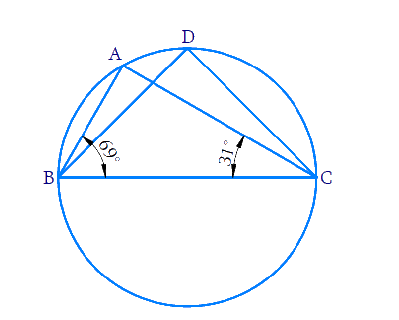### Solution

What is known?

Two angles in a triangle.

What is unknown?

Value of \begin {align} \angle {BDC}\end {align}

Reasoning:

• Sum of angles in a triangle is \begin {align} 180^{\circ}.\end {align}
• Angles in the same segment are equal.

Steps:

Consider the \begin {align} \Delta {ABC,}\end {align} the sum of all angles will be \begin {align} 180^\circ.\end {align}

\begin{align} \angle {ABC}+ \!\!\angle {BAC}+ \!\angle {ACB} &=180^{\circ} \\ 69^{\circ}+\angle {BAC}+31^{\circ} &=180^{\circ} \end{align}

\begin{align} \angle {BAC} &=180^{\circ}-\left(69^{\circ}+31^{\circ}\right) \\ &=180^{\circ}-100^{\circ} \\ &=80^{\circ} \end{align}

We know that, angles in the same segment of a circle are equal.

\begin {align} ∴ \angle {BDC}=\angle {BAC}=80^{\circ} \end {align}

## Chapter 10 Ex.10.5 Question 5

In the given figure, $$A, B, C$$ and $$D$$  are four points on a circle. $$AC$$ and $$BD$$  intersect at a point \begin {align}{E} \end {align} such that \begin {align} \angle {BEC}=130^{\circ} \end {align} and \begin {align} \angle {ECD}=20^{\circ}. \end {align} Find \begin {align} \angle {BAC}\end {align}.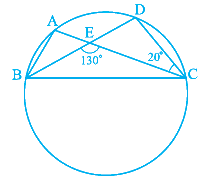### Solution

What is known?

Two angles  \begin {align} \angle {BEC}=130^{\circ} \end {align}

and \begin {align} \angle {ECD}=20^{\circ} \end {align}

What is unknown?

Value of  $$\angle {BAC}$$

Reasoning:

• Sum of angles in a triangle is $$180^{\circ}.$$
• Angles in the same segment are equal.

Steps:

Consider the straight line \begin {align} {BD} \end {align}. As the line \begin {align} {AC} \end {align} intersects with the line \begin {align} {BD,} \end {align} then the sum of two adjacent angles so formed is $$180^{\circ}.$$

Therefore,

\begin{align} \angle {BEC}+\angle {DEC} &=180^{\circ} \\ 130^{\circ}+\angle {DEC} &=180^{\circ} \\ \angle {DEC} &=180^{\circ}-130^{\circ} \\ &=50^{\circ} \end{align}

Consider the \begin{align} \Delta {DEC,} \end{align} the sum of all angles will be $$180^{\circ}.$$

\begin{align} \angle {DEC}+\angle {EDC}+\angle {ECD} &=180^{\circ} \\ 50^{\circ}+\angle {EDC}+20^{\circ} &=180^{\circ} \end{align}

\begin{align} \angle {EDC} &=180^{\circ}-70^{\circ} \\ &=110^{\circ} \\ ∴ \angle {BDC}=\angle {EDC} &=110^{\circ} \end{align}

We know that, angles in the same segment of a circle are equal.

\begin {align} ∴ \angle {BAC}=\angle {BDC}=110^{\circ}\end {align}

## Chapter 10 Ex.10.5 Question 6

\begin {align} {ABCD}\end {align} is a cyclic quadrilateral whose diagonals intersect at a point \begin {align} {E. }\end {align}If \begin {align} \angle {DBC}=70^{\circ}, \quad \angle {BAC}=30^{\circ} \end {align} find \begin {align} \angle {BCD}. \end {align} Further if \begin {align} {AB=BC,} \end {align} find \begin {align} \angle {ECD.} \end {align}

### Solution

What is known?

\begin {align} {ABCD}\end {align} is cyclic quadrilateral. \begin {align} {DBC}=70^\circ \end {align} and \begin {align} {BAC}=30^\circ. \end {align} \begin {align}{AB = BC.}\end {align}

What is unknown?

Value of \begin {align} \angle {BCD} \text { and } \angle {ECD} \end {align}

Reasoning:

• A quadrilateral \begin {align} {ABCD}\end {align} is called cyclic if all the four vertices of it lie on a circle.
• The sum of either pair of opposite angles of a cyclic quadrilateral is $$180^\circ.$$
• Sum of angles in a triangle is $$180^\circ.$$
• Angles in the same segment are equal.

Steps:

Based on the data given, draw the figure.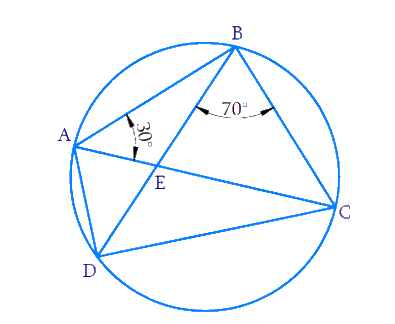In the triangles $${ABD}$$ and $${BCD,}$$ \begin {align} \angle {CAD}=\angle {CBD}=70^{\circ}. \end {align} (Angles in the same segment)

So \begin {align} \angle {BAD}=30^{\circ}+70^{\circ}=100^{\circ} \end {align}

Since \begin {align} {ABCD }\end {align}is a cyclic quadrilateral, the sum of either pair of opposite angles of cyclic quadrilateral is $$180^{\circ}.$$

\begin{align} \angle {BAD}+ \angle {BCD}&=180^{\circ} \\ \angle {BCD} &=180^{\circ}-100^{\circ} \\&=80^{\circ} \end{align}

Also given \begin{align} {AB = BC.}\end{align}

So, \begin {align} \angle {BCA}=\angle {BAC}=30^{\circ} \end {align} (Base angles of isosceles triangle are equal)

\begin{align} ∴ \quad \angle {ECD} &=\angle {BCD}-\angle {BCA} \\ &=80^{\circ}-30^{\circ} \\ &=50^{\circ} \end{align}

## Chapter 10 Ex.10.5 Question 7

If diagonals of a cyclic quadrilateral are diameters of the circle through the vertices of the quadrilateral, prove that it is a rectangle.

### Solution

What is known?

Diagonals of a cyclic quadrilateral are diameters of a circle passing through the vertices.

What is unknown?

To prove lines joining the vertices is a rectangle.

Reasoning:

• The angle subtended by an arc at the centre is double the angle subtended by it at any point on the remaining part of the circle.
• The sum of either pair of opposite angles of a cyclic quadrilateral is $$180^{\circ}.$$
• Diameter is a chord.

Steps: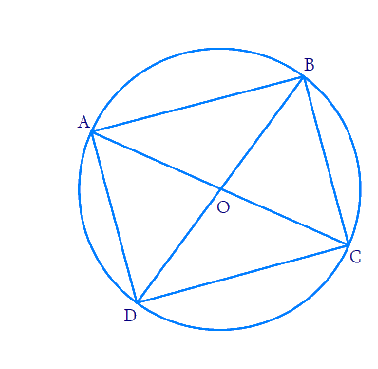Let $${DB}$$ be the diameter of the circle which is also a chord.

Then  \begin {align} \angle {BOD}=180^{\circ} \end {align}

We know that, the angle subtended by an arc at the centre is double the angle subtended by it at any point on the remaining part of the circle.

\begin{align} \angle {BAD} &=\frac{1}{2} \times \angle {BOD} \\ &=90^{\circ} \end{align}

Similarly,  \begin {align} \angle {BCD}=90^{\circ} \end {align}

Now considering $$\text{AC}$$ as the diameter of the circle, we get \begin {align} \angle {AOC}=180^{\circ} \end {align}

We know that, the angle subtended by an arc at the centre is double the angle subtended by it at any point on the remaining part of the circle.

\begin{align} \angle {ABC} &=\frac{1}{2} \times \angle {AOC} \\ &=90^{\circ} \end{align}

Similarly, \begin {align} \angle {ADC}=90^{\circ} \end {align}

As you can see, all the angles at the corners are $$90^\circ$$we can say that the shape joining the vertices is a rectangle.

This problem can also be solved by using the property of cyclic quadrilaterals.

## Chapter 10 Ex.10.5 Question 8

If the non-parallel sides of a trapezium are equal, prove that it is cyclic.

### Solution

What is known?

Non-parallel sides of trapezium are equal.

What is unknown?

To prove that trapezium is cyclic.

Reasoning:

If the sum of a pair of opposite angles of a quadrilateral is $$180^{\circ},$$ the quadrilateral iscyclic. Using Right angled-Hypotenuse-Side (RHS) criteria and Corresponding parts of congruent triangles (CPCT) we prove the statement.

Steps:

Draw a trapezium $${ABCD}$$

with $${AB} \| {CD}$$

$$AD$$ and $$BC$$ are the non-parallel sides which are equal. $${AD = BC.}$$

Draw \begin {align} {AM} \perp {CD} \end {align}

and \begin {align} {BN} \perp {CD} \end {align}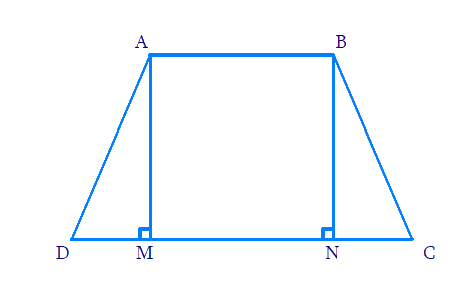Consider $$\Delta {AMD}$$ and $$\Delta \text{BNC.}$$

\begin{align} {AD} &={BC} \qquad \; (\text { Given }) \\\angle{AMD}&=\angle {BNC} \quad \left(90^{\circ}\right) \end{align}

$$AM =BN$$ (Perpendicular distance between two parallel lines is same)

By RHS congruence,

\begin {align}\Delta {AMD} \cong \Delta {BNC} \end {align}

Using CPCT ,

\begin {align} \angle {ADC}=\angle {BCD} \ldots . .(1) \end {align}

$$\angle {BAD}$$ and $$\angle {ADC}$$ are on the same side of transversal $$\angle \text{AD}.$$

\begin{align}\angle {BAD}+\angle {ADC}&=180^{\circ} \\ \angle {BAD}+\angle {BCD}&=180^{\circ} \text{[Using}(1)]\end{align}

This equation proves that the opposite angles are supplementary.

Hence, $${ABCD}$$ is a cyclic quadrilateral.

## Chapter 10 Ex.10.5 Question 9

Two circles intersect at two points $${B}$$ and $${C.}$$ Through $${B,}$$ two line segments $${ABD}$$ and $${PBQ}$$ are drawn to intersect the circles at $$A, D, P$$ and $$Q$$ respectively. Prove that \begin {align} \angle {ACP}=\angle {QCD.} \end {align}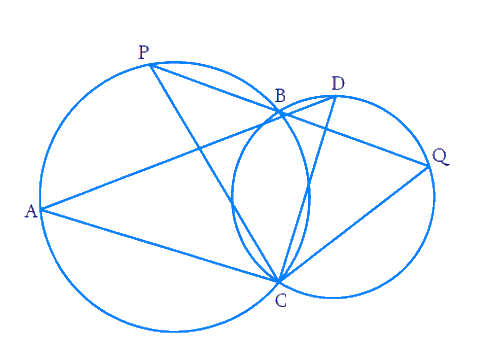### Solution

What is given ?

Two circles intersect at two points

What is unknown?

Proof of $$\angle {ACP}=\angle {QCD}$$

Reasoning:

$$\angle {ACP}$$  and  $$\angle {ABP}$$ lie on the same segment.

Similarly, $$\angle {DCQ}$$  and  $$\angle {DBQ}$$ lie on the same segment.

Angles in the same segment of a circle are equal.

Steps:

We know that, angles in the same segment of a circle are equal.

So we get  $$\angle {ACP}=\angle {ABP}$$  and  $$\angle {QCD}=\angle {QBD}$$

Also, \begin {align} \angle {QBD}=\angle {ABP} \end {align}(Vertically opposite angles)

Therefore $$\angle {ACP}=\angle {QCD}$$

## Chapter 10 Ex.10.5 Question 10

If circles are drawn taking two sides of a triangle as diameters, prove that the point of intersection of these circles lie on the third side.

### Solution

What is given ?

Two circles are drawn taking two sides of a triangle as diameters.

What is unknown?

To prove  that point of intersection of the $$2$$ circles lie on the third side.

Reasoning:

Angle in a semicircle is a right angle. By using this fact we can show that $$BDC$$ is a line which will lead to the proof that point of intersection lie on the third side.

Steps: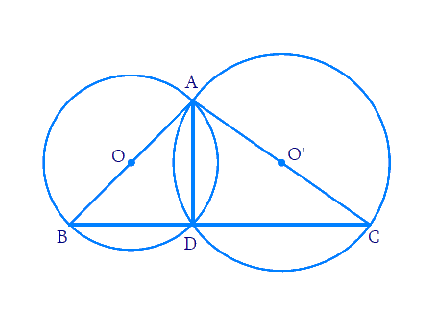Since angle in a semicircle is a right angle, we get:

\begin{align} \angle {ADB}&=90^{\circ} \text { and } \angle {ADC}=90^{\circ} \end{align}

\begin{align} \angle {ADB}+\angle {ADC}&=90^{\circ}+90^{\circ} \\ \Rightarrow \;\; \angle {ADB}+\angle {ADC}&=180^{\circ} \end{align}

$$\Rightarrow \quad{BDC} \text { is a straight line. }$$

∴ $${D}$$ lies on $${BC}$$

Hence, point of intersection of circles lie on the third side $$BC.$$

## Chapter 10 Ex.10.5 Question 11

$${ABC}$$ and $${ADC}$$ are two right triangles with common hypotenuse $${AC.}$$ Prove that $$\angle{CAD}= \angle {CBD}.$$

### Solution

What is given ?

$${ABC}$$ and $${ADC}$$ are two right triangles with common hypotenuse $${AC.}$$

What is unknown?

Proof of $$\angle{CAD}= \angle {CBD}$$

Reasoning:

Sum of all angles in a triangle is $$180^\circ$$

If the sum of pair of opposite angles in a quadrilateral is $$180$$ then it is cyclic quarilateral

Angles in the same segment of a circle are equal.

Steps: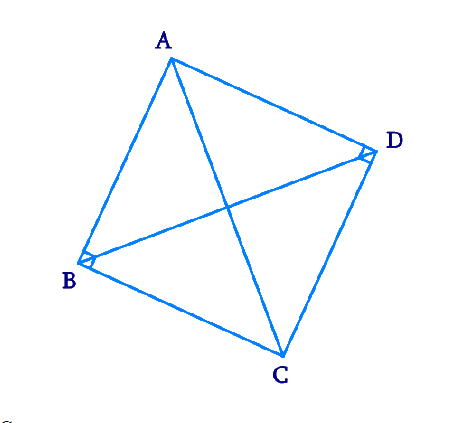Consider $$\Delta {ABC,}$$

\begin{align}&\angle {ABC}+ \angle {BCA}+\angle {CAB} =180^{\circ} \\&\text { (Angle sum property of a triangle) }\end{align}

\begin{align} 90^{\circ}+\angle {BCA}+\angle {CAB}&=180^{\circ} \\ \angle {BCA}+\angle {CAB}&=90^{\circ} \ldots(1)\end{align}

Consider $$\Delta {ADC,}$$

\begin{align}&\angle {CDA}+ \angle {ACD}+\angle {DAC} =180^{\circ} \\&\text { (Angle sum property of a triangle) }\end{align}

\begin {align} 90^{\circ}+\angle {ACD}+\angle {DAC}&=180^{\circ}\\\angle {ACD}+\angle {DAC}&=90^{\circ} \ldots(2) \end {align}

Adding Equations ($$1$$) and ($$2$$), we obtain

\begin {align} \begin{Bmatrix} \angle {BCA}+\angle {CAB}\\+\angle {ACD}+\angle {DAC} \end{Bmatrix}&=180^{\circ} \\ \begin{Bmatrix} (\angle {BCA}+\angle {ACD})+ \\ (\angle {CAB}+\angle {DAC}) \end{Bmatrix} &=180^{\circ} \end {align}

\begin {align} \angle {BCD}+\angle {DAB}&=180^{\circ} \ldots(3) \end {align}

However, it is given that

\begin {align}\angle {B}+\angle {D} &=90^{\circ}+90^{\circ} \\ &=180^{\circ} \ldots(4) \end {align}

From Equations ($$3$$) and ($$4$$), it can be observed that the sum of the measures of opposite angles of quadrilateral $$\text{ABCD}$$ is $$180^{\circ}.$$ Therefore, it is a cyclic quadrilateral.

Consider chord $${CD.}$$

\begin {align}\angle {CAD} = \angle {CBD} \end {align} (Angles in the same segment)

## Chapter 10 Ex.10.5 Question 12

Prove that cyclic parallelogram is a rectangle.

### Solution

What is given ?

What is unknown?

Prove  that cyclic parallelogram is a rectangle.

Reasoning:

The sum of either pair of opposite angles of a cyclic quadrilateral is $$180^\circ.$$ By using this fact we can show each angle of cyclic parallelogram as $$90^\circ$$ which will prove the statement it is a rectangle.

Steps: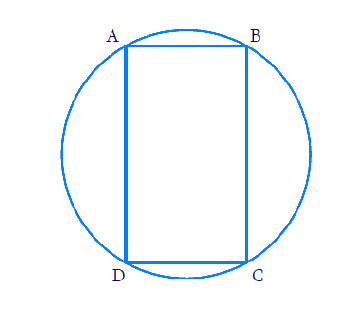Let $${ABCD}$$ be the cyclic parallelogram.

We know that opposite angles of a parallelogram are equal.

\begin {align}\angle {A}=\angle {C} \text { and } \angle {B}=\angle {D} \ldots .(1) \end {align}

We know that the sum of either pair of opposite angles of a cyclic quadrilateral is $$180^{\circ}.$$

\begin {align} \angle {A}+\angle {C}=180^{\circ} \ldots .(2) \end {align}

Substituting ($$1$$) in ($$2$$),

\begin{align}\angle {A}+\angle {C}&=180^{\circ} \\ \angle {A}+\angle {A}&=180^{\circ} \\ 2 \angle {A}&=180^{\circ} \\ \angle {A}&=90^{\circ}\end{align}

We know that if one of the interior angles of a parallelogram is $$90^{\circ},$$ all the other angles will also be equal to $$90^{\circ}.$$

Since all the angles in the parallelogram is $$90^{\circ},$$ we can say that parallelogram $${ABCD}$$ is a rectangle.

Related Sections
Related Sections
Instant doubt clearing with Cuemath Advanced Math Program